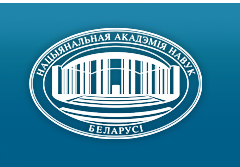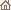Home News About Academy Academy Activities Academy Membership Organizations Publications Conferences Contacts Site Map

# Proc. of the Natl. Academy of Sciences of Belarus, Ser. Phys.-Math. Sci., 2009, No.1/ Publications / Scientific Journals

### Number 1, 2009## CONTENTS

### MATHEMATICS

Kharin Yu. S., Voloshko V. A.
One method of robust estimation of autoregressive parameters under outliers. pp. 4--12

Summary: The problem of statistical estimation of coefficients of an autoregressive time series of order p in the presence of random outliers with a symmetric probability distribution is considered. A new method of parametric robust estimation of the correlation function and coefficients for the autoregressive process is proposed. Asymptotic properties of these estimators are analysed. Numerical results on comparison of the proposed method with the Huber estimator and the LS-estimator are given.

Troush N. N., Le Hong Son
Estimator of parameters of GARCH(1,1) model with stable innovations. pp. 13--21

Summary: The M-estimator of the parameters of the models GARCH(1,1) with stable innovations is constructed. The consistency and the asymptotic normality of the M-estimator in the class of the above models are given.

Tanyhina A. N.
Analogs of the LeChatelier--Samuelson principle for the open Leontiev--Ford model. pp. 22--28

Summary: The article is devoted to the LeChatelier -- Samuelson principle for the open Leontiev -- Ford model. The analogs of this principle are proved both in the case, when r(A) < 1 for the technological matrix A of the model, and in the case, when r(A) ³ 1.

Savelyeva N. V., Vorob'ev N. T.
Problem of existence of maximal subclasses of the minimal p-normal Fitting class. pp. 29--37

Summary: It is proved that there are no nontrivial maximal local Fitting subclasses in the minimal p-normal Fitting class (Gp)* where p is a nonempty set of primes.

Korzyuk V. I., Shushkevich G. Ch.
Shielding of a magnetic field by a system of screens: thin open-ended ellipsoidal shell and spherical shell. pp. 38--46

Summary: The solution of the problem of nonaxially symmetric magnetic field penetration into an ideally thin open-ended ellipsoidal shell in the presence of a spherical shell is reduced to the solution of an infinite system of linear algebraic equations of the second kind. The effect of the apex angle of the open-ended ellipsoidal shell, geometric parameters of the screens and electro-physical properties of the spherical shell material on the field attenuation coefficient inside the ellipsoidal shell is studied numerically.

Gaiduk S. I., Kuleshov A. A.
One mixed problem of the theory of beam oscillations. pp. 47--51

Summary: A solution of one problem on kinematic impact-caused transverse oscillations of a homogeneous elastic beam at one point described by the Timoshenko system of equations is constructed and substantiated.

Shwed O. L.
Determination of the elastic spin tensor in the nonlinear theory of plasticity. pp. 52--58

Summary: With the demands of the potentiality of the governing equations the spin of a proper orthogonal tensor following the elastic deformation in the active process is generated in strain speeds. The introduced relations can be used for developing computer systems of numerical modeling of deformable metal bodies.

### PHYSICS

Maksimenko N. V., Timoshin E. S.
Spin structure of the nucleon in the theory of electroweak interaction. pp. 59--63

Summary: The contributions of the quark flavours (u, d, s) and the valence quarks in the nucleon spin have been expressed through the first moments of the polarized structure functions of deep inelastic lepton-nucleon scattering with neutral current taking into account weak interaction.

Baryshevsky V. G., Rouba A. A.
Influence of the Coulomb interaction on the deuteron spin dichroism effect in the energy range 5--20 MeV. pp. 64--69

Summary: When an unpolarized deuteron beam passes through an unpolarized target, the tensor polarization of beam arises due to deuteron spin dichroism. It is shown, that the interference of Coulomb and nuclear interactions affects essentially the value and the behavior of deuteron spin dichroism in the energy range 5--20 MeV. Qualitative agreement between theoretical and experimental results is obtained.

Ryabushko A. P., Zhur T. A.
Relativistic effects of the motion of a body in the gravitational field of a heterogeneous medium. II. The post-Newtonian approximation of the general theory of relativity. pp. 70--75

Summary: In the post-Newtonian approximation of the general theory of relativity the relativistic energy integral, the orbital angular momentum and the equation of the orbit of a test body in the medium have been derived and discussed. Several relativistic effects are predicted: an additional acceleration of the body as a systematic origin to Pioneer anomaly, an inverse displacement of the perihelion, the shortening of the orbit and an increase of the pulsation of the sectorial and orbital velocity of the motion of a body. Some numerical values of these effects for the Mercury, Earth and Pluto orbits are given.

Makarenko L. F.
Image potential influence on the ionization energy of a hydrogen-like center near the interface of two media. pp. 76--81

Summary: This article considers the application of simple trial wave functions to calculate the ground state energy of a hydrogen-like center near the interface of two media. Calculations have been performed taking into account the image potential. It has been shown that different kinds of wave functions are optimal at different distances from the interface. A relatively simple wave function has been suggested to represent main features of the dependence of the ground state energy on the distance to the interface.

Tsymbarevich Y. G.
To the theory of radiation transfer in scattering strongly fluctuating media. pp. 82--88

Summary: A small-angle iteration method of analytical approximate solving of a stochastic equation of transfer is described. This method is valid for any statistics of a medium and strongly anisotropic scattering. Its convergence and accuracy are studied for a binary Markovian mixture. The method is shown to be applicable for description of radiation transfer in media with strongly fluctuating optical properties.

Nepokojchitskij A. G., Ignatov B. I., Frantskevich K. V., Astashenko S. G.
Some peculiarities of formation of metal coatings on metal-oxide compositions. pp. 89--92

Summary: Some regularities of formation of metal coatings on metal-oxides and ferrite under the influence of electrochemical and electroplasmic processes are viewed. A method of obtaining coatings of any shape and size is suggested. It is applicable in radio and electrotechnics, electronics, calculation techniques for production of passive LRC elements, current conductor channels on the surface and inside oxide materials and for other purposes.

Kalanda N. A., Kochonovski L. V., Pavlenko A. A.
Desorption dynamics and oxygen nonstoichiometry in La0.6Sr0.4МnO3-d. pp. 93--97

Summary: On the basis of the obtained results it is established that the rate of oxygen evolution from La0.6Sr0.4МnO3-d is the function of the partial pressure of oxygen (pO2). It is assumed that this behavior of the activation energy of oxygen diffusion is caused by the presence of the stressed layer on the grain surface. During the oxygen evolution from La0.6Sr0.4МnO3-d there are two minimums of the current of titration caused by the bond cleveage of anions with trivalent and tetravalent manganese, and also by the formation of the transition layer located near the surface of the grain depleted with oxygen and simultaneously being a buffer for diffusing oxygen from the grain.

Khapalyuk A. P.
Solution of diffraction-type Maxwell equations. pp. 98--107

Summary: A precise analytical solution of the diffraction problem on the ideal flat screen of a rather general view is obtained. The problem is formulated as a boundary-value problem on Maxwell equations. A diffracting wave and diffraction waves are precise solutions of Maxwell equations, which are determined by tangential vector components of the wave field on the screen as arbitrary functions of the coordinates of the screen plane. Therefore, a diffracting wave can be arbitrary (not necessarily flat) and electrodynamic properties of the screen, which determine diffraction waves, can be arbitrary. The precise analytical solutions are represented as integrals, that are uniquely determined through the boundary functions given in the problem at statement.

### INFORMATICS

Likhoded N. A.
Preserving the dependences between operations at parallelizing algorithms. pp. 108--112

Summary: Conditions for preservation of dependences when parallelizing of algorithms are obtained. Unlike the other methods which based on the Farkash lemma, the method can be applied for arbitrary and beforehand unknown values of external variables.

Benediktovitch V. I.
Distinctive number of the geometric clique К7. pp. 113--118

Summary: In this article a distinctive number of geometric clique K7 is found.

### SHORT NOTES

Shamukova N V.
Relationship between the values of integer polynomial coefficients and their roots in the fields of complex and p-adic numbers pp. 119--121

Summary: In the article it was shown how by means of linear-fractional transformations of argument any integer polynomial can be reduced to the polynomial with the values of roots and p-adic norm not larger than some value that doesn't depend on the height of polynomial.

### SCIENTISTS OF BELARUS

Vladimir Arkhipovich Labunov (To the 70th Anniversary of Birthday). pp. 122--123
Click
here to read this article in Russian (Win 1251)

Valentin Vikentievich Gorokhovik (To the 60th Anniversary of Birthday). pp. 124--125
Click here to read this article in Russian (Win 1251)/ Publications / Scientific Journals / Back to Top of Page

Designed and maintained by Dr. Nikolai N. Kostyukovich. Last updated: June 10, 2009
Created with assistance of Dr. Ignatii I. Korsak
Copyright © 2009 The National Academy of Sciences of Belarus
Copyright © 2009 The Belaruskaya Navuka Publishing House